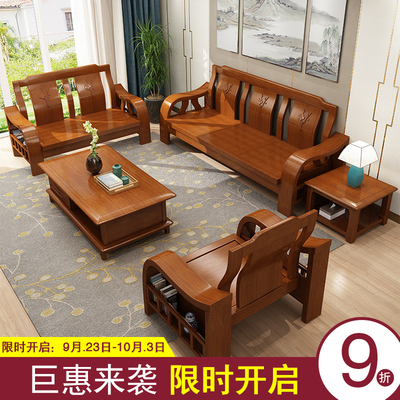群英柏亿 QUNYINGBAIYI6602实体店，群英柏亿实木沙发质量好不好• 品牌:群英柏亿 QUNYINGBAIYI
• 型号:6602
• 图案:艺术
• 风格:现代中式
• 适用人数:组合
• 颜色分类:1+1+31+1+3+长茶几+方茶几1+2+31+2+3+长茶几+方茶几2+2+32+2+3+长茶几+方茶几三人位1+1+3+坐垫1+1+3+长茶几+方茶几+坐垫1+2+3+坐垫1+2+3+长茶几+方茶几+坐垫2+2+3+坐垫2+2+3+长茶几+方茶几+坐垫三人位+坐垫长茶几方茶几
• 毛重:400
• 饰面材质:其他
• 填充物:海绵
• 饰面工艺:油漆工艺
• 饰面油漆工艺:喷漆
• 是否可定制:否
• 沙发组合形式:其他
• 是否可拆洗:是
• 附加功能:移动
• 适用对象:成人
• 是否带储物空间:是
• 木质材质:橡胶木
• 木质结构工艺:榫卯结构
• 可送货/安装:其他北京南昌市大连市沈阳市西安市贵阳市郑州市金华市长沙市常州市成都市东莞市佛山市福州市广州市杭州市南京市南宁市宁波市青岛市上海深圳市苏州市台州市天津温州市无锡市武汉市厦门市重庆
• 产地:江西省
• 地市:赣州市
• 区县:南康市
• 是否组装:组装
• 包装体积:1.5
• 适用人群:成人
• 是否可预售:否
• 出租车是否可运输:否
• 设计元素:其他卯榫原木大师设计异形拼接雕花
• 款式定位:艺术风格型
• 安装说明详情:提供简单安装工具